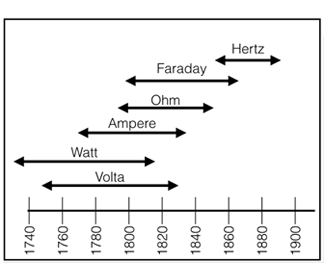# Electrical HistoryCurrent
Symbol is I for Intensity
Measured in Amperes, colloquially Amps
Hydraulic analogy = gallons per hour
Meter used to measure current is usually called an Ammeter
Named after the French physicist Andre Ampere

Voltage
Symbol is V for Voltage or Volts, or E for Electromotive Force (EMF)
Measured in Volts, abbreviated V
Hydraulic analogy = water pressure
Named after the Italian physicist Alessandro Volta

Resistance
Symbol is R for Resistance
Measured in Ohms, abbreviated with the omega symbol
Named after the German physicist Georg Simon Ohm

Power
Symbol is P
Measured in Watts, abbreviated W
Named after the Scottish engineer James Watt

Joule's law
P=VI for DC
P=VIK for AC where K is the power factor from +1 to -1
Named after the British physicist James Joule

Galvanometer
The original Ammeter
Named after Luigi Galvani who had the concept of animal electricity, later disproved.

Guglielmo Marconi
Wireless transmission between the US and Great Britain January 18, 1903.

Kirchhoff's Current Law
The sum of currents entering and leaving a node equals zero.
Named after German physicist Gustav Robert Kirchhoff (1824-1887), a student of Gauss.

Kirchhoff's Voltage Law
The sum of branch Voltages around a loop equals zero.
Named after German physicist Gustav Robert Kirchhoff (1824-1887), a student of Gauss.

Gauss
The unit of measurement of magnetic strength.
Named after German scientist Carl Friedrich Gauss (1777-1855)

Frequency
Symbol is Hz. Prior to 1968 it was called cycles per second and the symbol was cps.
Named after the German physicist Heinrich Hertz (1857-1894)